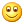# 亲子教育YY群学员博然接受指导的经过及进步的历程（二）

by on 五月 23, 2011 ·*~*紫雨老师*~* (11:20:46)

*~*紫雨老师*~* (11:22:12)

*~紫雨老师~* (17:45:13)

*~紫雨老师~* (17:47:16)

*~紫雨老师~* (17:48:09)

*~紫雨老师~* (17:51:08)

*~紫雨老师~* (17:51:42)

*~紫雨老师~* (17:57:29)

*~紫雨老师~* (17:57:51)

*~紫雨老师~* (17:58:17)

*~紫雨老师~* (17:59:18)

*~紫雨老师~* (17:59:30)

*~紫雨老师~* (17:59:45)

*~紫雨老师~* (18:00:01)

*~紫雨老师~* (18:01:02)

*~紫雨老师~* (18:01:34)

*~紫雨老师~* (18:01:57)

*~紫雨老师~* (18:02:11)

*~紫雨老师~* (18:03:13)有进步哦！

知道吗？我看到的，是你在开始进步了！*~紫雨老师~* (02:56:34)

*~紫雨老师~* (19:27:39)

# 亲子教育YY群学员博然接受指导的经过及进步的历程（一）{ 2 comments… read them below or add one }木子清华 五月 25, 2011 5:05 下午黑咖啡 五月 29, 2011 1:03 下午

Previous post:

Next post: